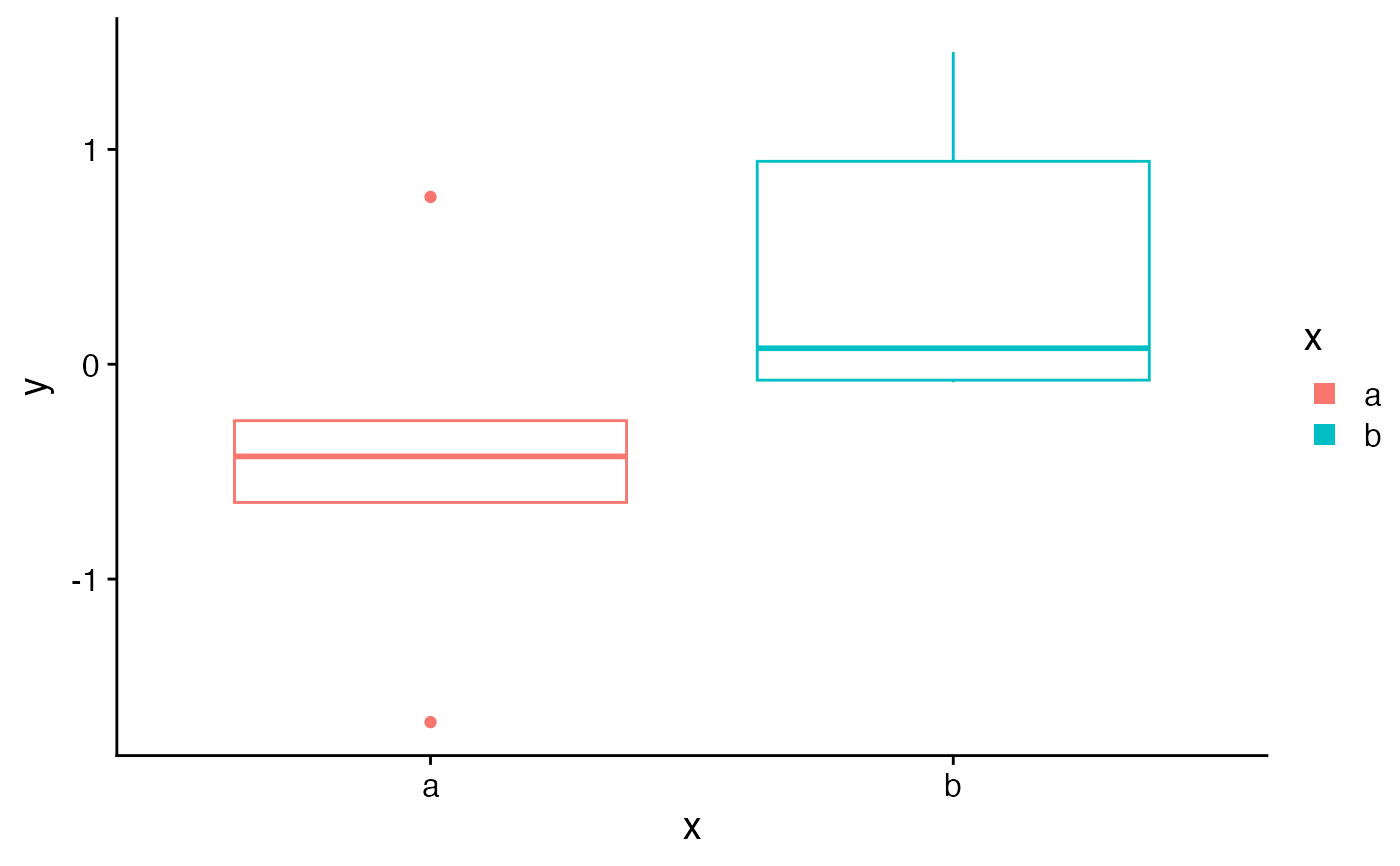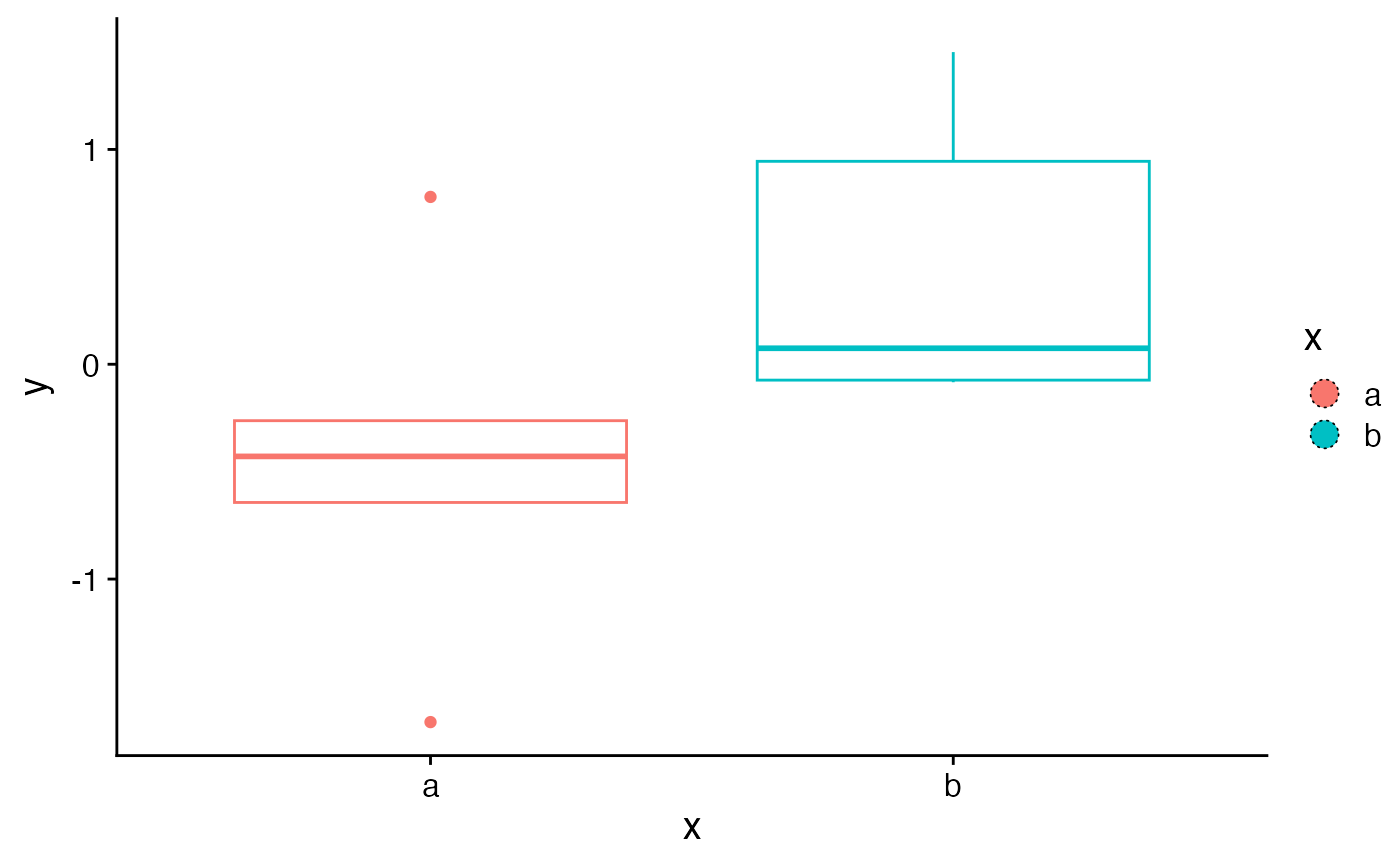These functions create customizable legend key glyphs, such as filled rectangles or circles.

rectangle_key_glyph(colour = NA, fill = fill, alpha = alpha,
size = size, linetype = linetype, padding = unit(c(0, 0, 0, 0),
"pt"), color)

circle_key_glyph(colour = NA, fill = fill, alpha = alpha,
size = size, linetype = linetype, padding = unit(c(0, 0, 0, 0),
"pt"), color)

## Arguments

colour, color Unquoted name of the aesthetic to use for the outline color, usually colour, color, or fill. Can also be a color constant, e.g. "red". Unquoted name of the aesthetic to use for the fill color, usually colour, color, or fill. Can also be a color constant, e.g. "red". Unquoted name of the aesthetic to use for alpha, usually alpha. Can also be a numerical constant, e.g. 0.5. Unquoted name of the aesthetic to use for the line thickness of the outline, usually size. Can also be a numerical constant, e.g. 0.5. Unquoted name of the aesthetic to use for the line type of the outline, usually linetype. Can also be a constant, e.g. 2. Unit vector with four elements specifying the top, right, bottom, and left padding from the edges of the legend key to the edges of the key glyph.

## Examples

library(ggplot2)

set.seed(1233)
df <- data.frame(
x = sample(letters[1:2], 10, TRUE),
y = rnorm(10)
)

ggplot(df, aes(x, y, color = x)) +
geom_boxplot(
key_glyph = rectangle_key_glyph(fill = color, padding = margin(3, 3, 3, 3))
)ggplot(df, aes(x, y, color = x)) +
geom_boxplot(
key_glyph = circle_key_glyph(
fill = color,
color = "black", linetype = 3, size = 0.3,
padding = margin(2, 2, 2, 2)
)
)